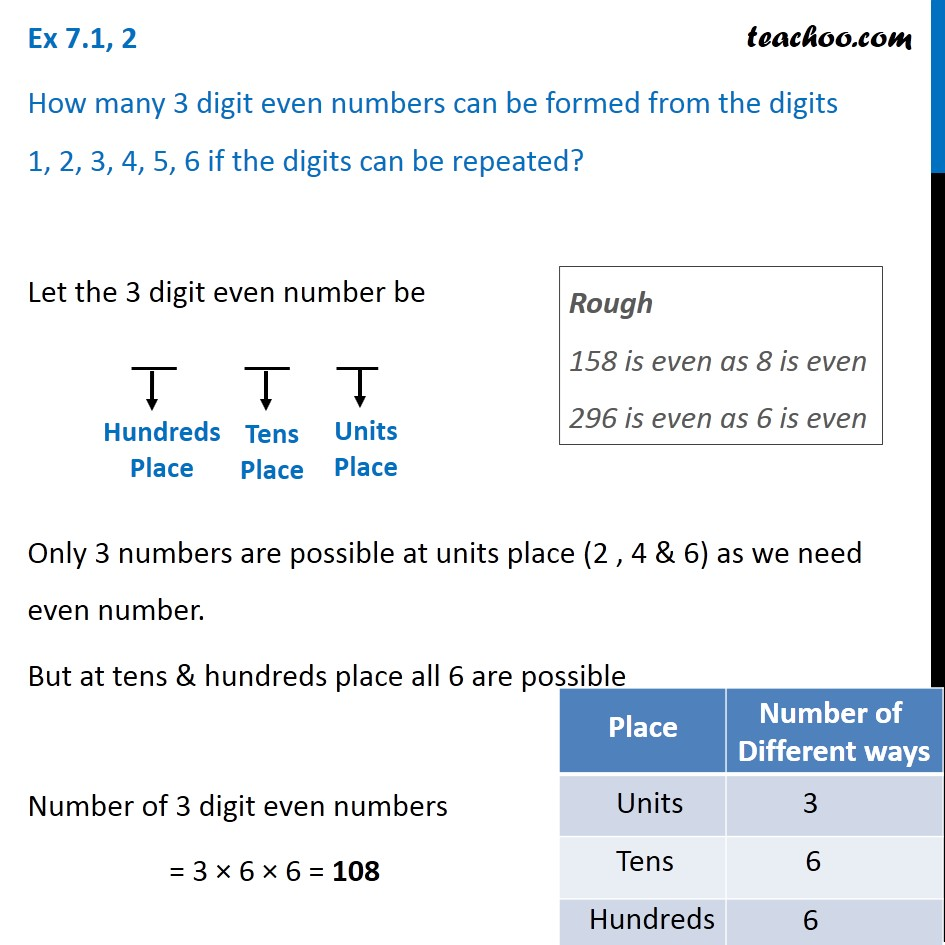Ex 6.1

Chapter 6 Class 11 Permutations and Combinations
Serial order wiseLearn in your speed, with individual attention - Teachoo Maths 1-on-1 Class

### Transcript

Ex 6.1, 2 How many 3 digit even numbers can be formed from the digits 1, 2, 3, 4, 5, 6 if the digits can be repeated? Let the 3 digit even number be Only 3 numbers are possible at units place (2 , 4 & 6) as we need even number. But at tens & hundreds place all 6 are possible Number of 3 digit even numbers = 3 × 6 × 6 = 108 Rough 158 is even as 8 is even 296 is even as 6 is even